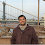## Friday, January 6, 2012

### Cost-Sensitive Binary Classification and Active Learning

Now that there are multiple active learning algorithms for binary classification popping up, it's a good time to ask what machine learning reductions can say about active learning for other types of learning problems. Right now I'll focus on cost-sensitive binary classification, for which the goal is to compete with $h^* = \mathop{\operatorname{arg\,min\;}}_{h \in H} E_{(X, Y, \Upsilon) \sim D}[ \Upsilon 1_{h (X) \neq Y}],$ where $X$ are features, $Y \in \{ 0,1 \}$ are labels, $\Upsilon \in [0, c_{max}]$ are costs, and $D$ is some unknown distribution.

#### Costs are visible

First I'll assume that we can see the costs in unlabeled data, i.e., without querying the label. This is not typical in practice, since the cost often depends upon the label (e.g., false positives are more expensive than false negatives). However this will provide some intuition about what is possible.

I can use rejection sampling to reduce cost-sensitive classification to binary classification and then apply an active learning binary classifier. Specifically, I can rejection sample the input stream with acceptance probability $\Upsilon_k / c_{max}$, and if the sample is accepted it discards the cost and uses binary classification. This is a regret transform, which scales the induced binary regret by $E[\Upsilon]$. Therefore if we have a high probability regret bound $r_b (n)$ in the underlying active binary classifier given $n$ examples, then presumably replacing $n \rightarrow (E[\Upsilon] / c_{max}) n + O (\frac{1}{n} \log \frac{1}{\delta})$ and scaling by $E[\Upsilon]$ will give us a high probability bound $r_c (n)$ on the regret in the original problem, $r_c (n) = E[\Upsilon] r_b \left( \frac{E[\Upsilon]}{c_{max}} n + O (\frac{1}{n} \log \frac{1}{\delta}) \right).$
Meanwhile, in the case of a rejection we definitely do not query the label $Y_k$. In the case of a non-rejection we might query the label $Y_k$ depending upon the probability of using the label in the induced active binary classifier. If we have a high probability bound on the label complexity of the induced binary classifier $l_b (n)$ given $n$ examples, then presumably replacing $n \rightarrow (E[\Upsilon] / c_{max}) n + O (\frac{1}{n} \log \frac{1}{\delta})$ will give a high probability bound on the label complexity $l_c (n)$ on the original cost-sensitive problem, $l_c (n) = l_b \left( \frac{E[\Upsilon]}{c_{max}} n + O (\frac{1}{n} \log \frac{1}{\delta}) \right).$ Here's a meta-algorithm for active learning with cost-sensitive binary classification with visible costs.
Algorithm:Visible costs case
Rejection sampling for active cost-sensitive binary classification with visible costs and active learning binary oracle $\mathcal{O}$.
1. Obtain unlabeled data point $X_k$ with cost $\Upsilon_k$.
2. Toss a biased coin with $\mathrm{Pr} (\mathrm{heads}) = (\Upsilon_k / c_{max})$.
1. If heads, pass unlabeled features $X_k$ to $\mathcal{O}$.
2. If tails, discard unlabeled data point.

If the binary oracle uses Agnostic Active Learning without Constraints, this reasoning suggests a regret bound like $\mathrm{err}_c (h_n) \leq \mathrm{err}_c (h^*) + O \left(\sqrt{c_{max} E[\Upsilon] \frac{\log n}{n}}\right)$ is possible, where $\mathrm{err}_c (h) = E_{(X, Y, \Upsilon) \sim D}[ \Upsilon 1_{h (X) \neq Y}],$ and a label complexity like $\mbox{labels requested} \leq 1 + \theta \cdot \frac{2}{c_{max}} \mathrm{err}_c (h^*) n + O\left( \theta \sqrt{\frac{E[\Upsilon]}{c_{max}} n \log n} \right)$ is possible. $\theta$ here is the disagreement coefficient for the induced binary subproblem.

#### Costs are not visible

Now I'll assume that the cost is only available if the label is queried. Suppose we decided to implement the above rejection sampling procedure by first accepting or rejecting according to the underlying active learning algorithm, and then if the label is queried and the cost $\Upsilon_k$ observed, flipping another coin and accepting with probability $(\Upsilon_k / c_{max})$. This would be consistent with the invisible costs constraint, but identical in terms of outcome. While this would be silly if the costs were visible, it is a feasible strategy if the costs are not visible. The regret bound would still be the same but now unfortunately the label complexity of the induced binary problem passes through unmodified, $l_c (n) = l_b (n)$. Of course, label complexity is the game in active learning, otherwise we would query every label and get the gold-standard passive learning regret bound. So having a worse label complexity for a particular regret bound is highly undesirable.

Algorithm:Invisible costs case
Rejection sampling for active cost-sensitive binary classification with invisible costs and active learning binary oracle $\mathcal{O}$.
1. Obtain unlabeled data point $X_k$.
2. Pass unlabeled data point $X_k$ to $\mathcal{O}$ and intercept the decision $Q_k \in \{ 0, 1 \}$ to query the label.
1. If $Q_k = 0$, do nothing.
2. If $Q_k = 1$,
1. Query the label and observe $\Upsilon_k$ and $Y_k$.
2. Toss a biased coin with $\mathrm{Pr} (\mathrm{heads}) = (\Upsilon_k / c_{max})$.
1. If heads, pass label $Y_k$ to $\mathcal{O}$ as is expected when $Q_k=1$.
2. If tails, discard label and undo'' the presentation of $X_k$ to $\mathcal{O}$.

In particular for a base learner utilizing Agnostic Active Learning without Constraints, this reasoning suggests when costs are not visible, $\mbox{labels requested} \leq 1 + \theta \cdot \frac{2}{E[\Upsilon]} \mathrm{err}_c (h^*) n+ O \left(\theta \sqrt{n \log n} \right).$ Comparison of the two label complexities suggests we might gain something like a $(E[\Upsilon] / c_{max})$ improvement in the non-realizable case between a smart'' cost-sensitive active learning algorithm and the dumb'' one sketched here.

#### 1 comment:

1.How do you get the term n \rightarrow (E[\Upsilon] / c_{max}) n + O (\frac{1}{n} \log \frac{1}{\delta}) in the third para ?